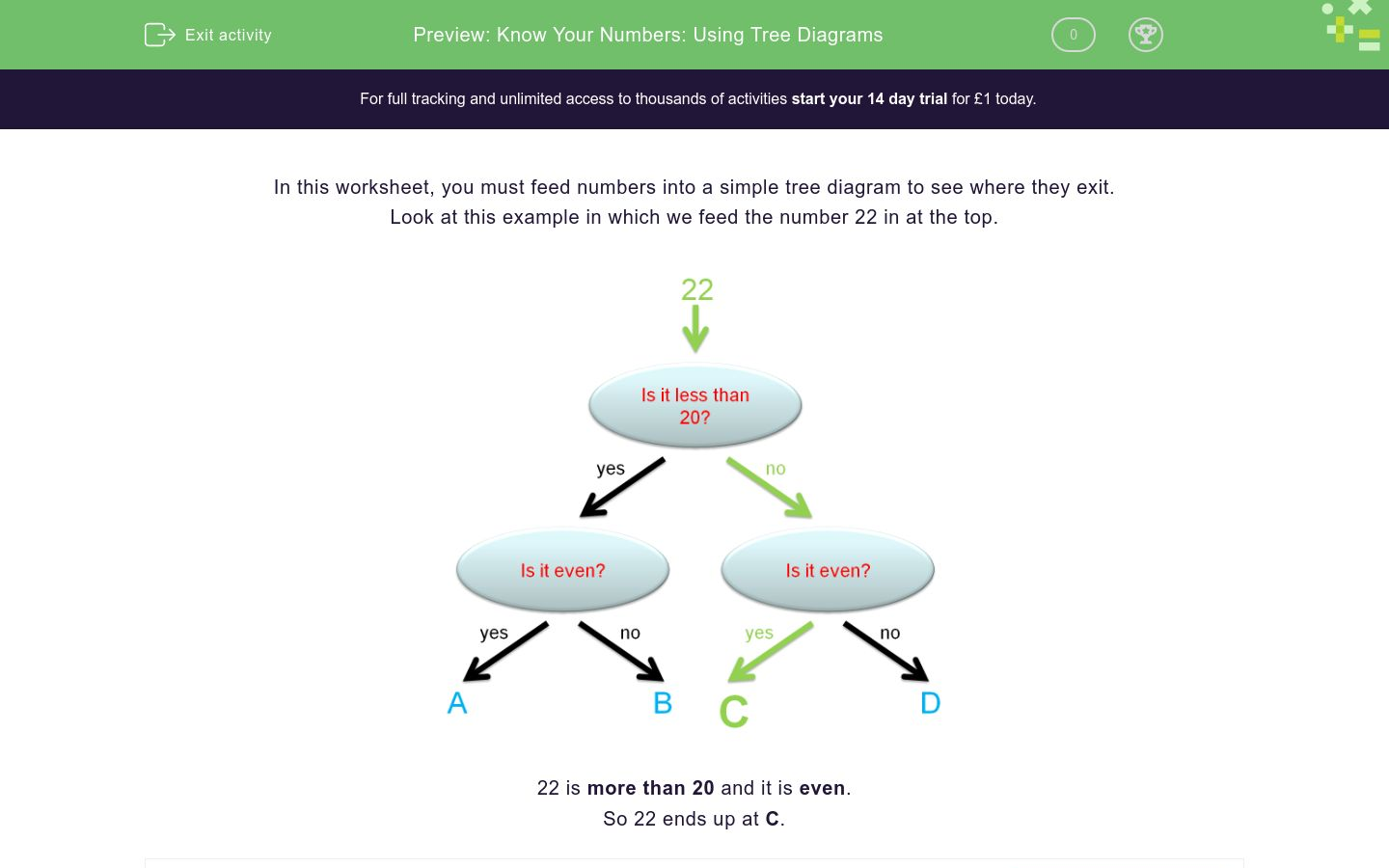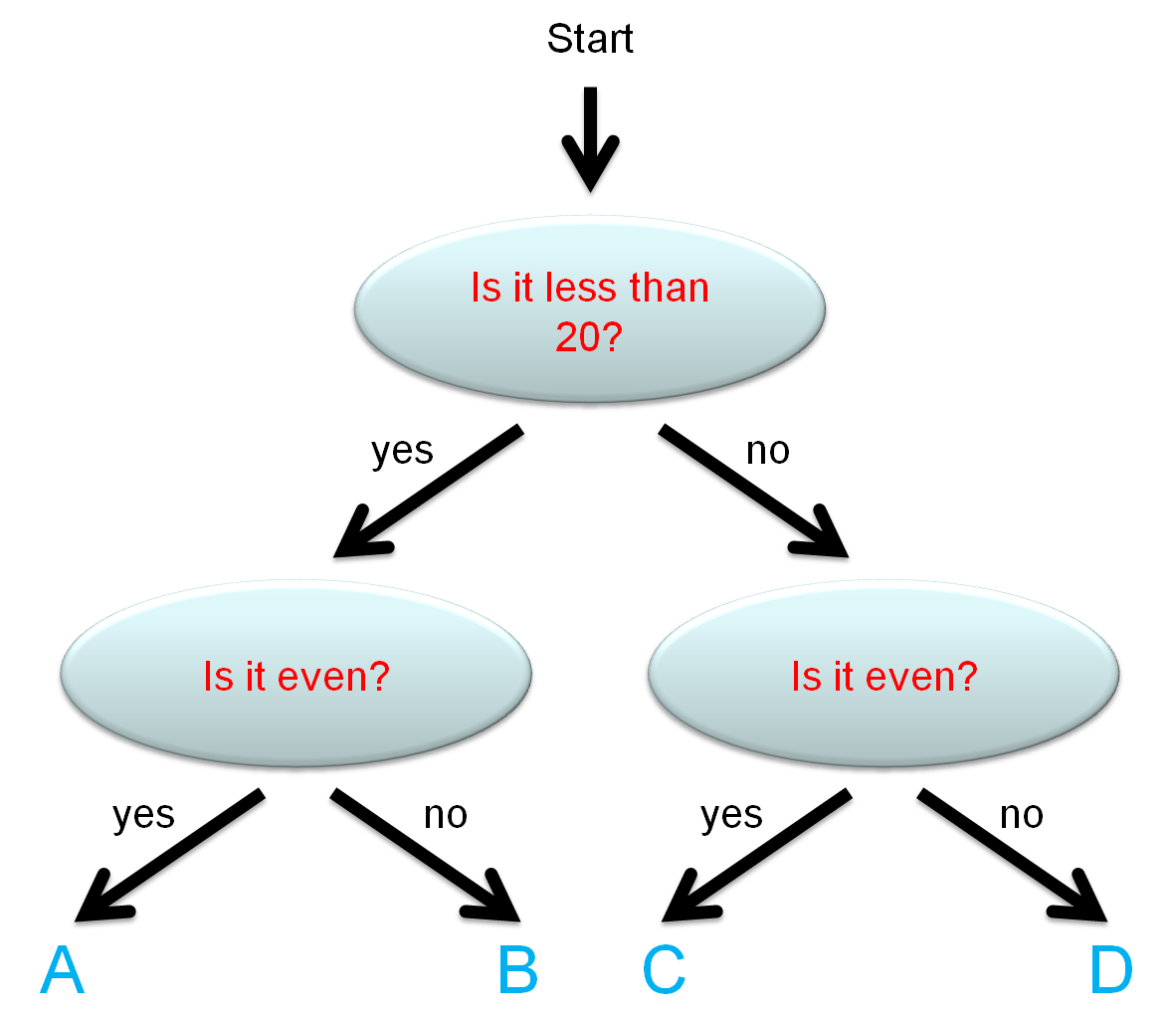# Know Your Numbers: Using Tree Diagrams

In this worksheet, students count numbers and read instructions to help them answer questions.Key stage:  KS 1

Curriculum topic:   Number: Number and Place Value

Curriculum subtopic:   Represent Numbers to 100

Difficulty level:### QUESTION 1 of 10

In this worksheet, you must feed numbers into a simple tree diagram to see where they exit.

Look at this example in which we feed the number 22 in at the top.22 is more than 20 and it is even.

So 22 ends up at C.

Put the number 24 into this tree diagram.

Where does it exit?A

B

C

D

Put the number 25 into this tree diagram.

Where does it exit?A

B

C

D

Put the number 14 into this tree diagram.

Where does it exit?A

B

C

D

Put the number 15 into this tree diagram.

Where does it exit?A

B

C

D

Put the number 21 into this tree diagram.

Where does it exit?A

B

C

D

Put the number 28 into this tree diagram.

Where does it exit?A

B

C

D

Put the number 30 into this tree diagram.

Where does it exit?A

B

C

D

Put the number 17 into this tree diagram.

Where does it exit?A

B

C

D

Put the number 18 into this tree diagram.

Where does it exit?A

B

C

D

Put the number 32 into this tree diagram.

Where does it exit?A

B

C

D

• Question 1

Put the number 24 into this tree diagram.

Where does it exit?C
• Question 2

Put the number 25 into this tree diagram.

Where does it exit?D
• Question 3

Put the number 14 into this tree diagram.

Where does it exit?A
• Question 4

Put the number 15 into this tree diagram.

Where does it exit?B
• Question 5

Put the number 21 into this tree diagram.

Where does it exit?D
• Question 6

Put the number 28 into this tree diagram.

Where does it exit?C
• Question 7

Put the number 30 into this tree diagram.

Where does it exit?C
• Question 8

Put the number 17 into this tree diagram.

Where does it exit?B
• Question 9

Put the number 18 into this tree diagram.

Where does it exit?A
• Question 10

Put the number 32 into this tree diagram.

Where does it exit?C
---- OR ----

Sign up for a £1 trial so you can track and measure your child's progress on this activity.

### What is EdPlace?

We're your National Curriculum aligned online education content provider helping each child succeed in English, maths and science from year 1 to GCSE. With an EdPlace account you’ll be able to track and measure progress, helping each child achieve their best. We build confidence and attainment by personalising each child’s learning at a level that suits them.

Get started